Assessing the impact on optimal production capacities in ...

Assessing the impact on optimal production capacities in a closed-loop logistics system of the assumption that returns are stochastically independent of sales

Ernesto Benedito, Albert Corominas

Universitat Politècnica de Catalunya (SPAIN)

Accepted September 2011

Benedito, E.; & Corominas, A. (2011). Assessing the impact on optimal production capacities in a closed-loop logistics system of the assumption that returns are stochastically independent of sales. Journal of Industrial Engineering and Management, 4(3), 504-522. http://dx.doi.org/10.3926/jiem.2011.v4n3.504-522

---------------------

Abstract:

Purpose: This paper is concerned with a reverse logistic system where returns are stochastically dependents on sales. The aim of the paper is to assess the influence on optimal production capacities when is assumed that returns are stochastically independent of sales.

Design/methodology/approach: This paper presents a model of the system. An approximated model where is assumed that returns are stochastically independent of sales, is formulated to obtain the optimal capacities. The optimal costs of the original and the approximated models are compared in order to assess the influence of the assumption made on returns.

Findings: The assumption that returns are stochastically independent of sales is significant in few cases.

Research limitations/implications: The impact of the assumption on returns is assessed indirectly, by comparing the optimal costs of both models: the original and approximated.

Practical implications: The problem of calculating the optimal capacities in the original model is hard to solve, however in the approximated model the problem is tractable. When the impact of the assumption that returns are stochastically independent of sales is not significant, the approximated model can be used to calculate the optimal capacities of the original model.

Originality/value: Prior to this paper, few papers have addressed with the problem of calculating the optimal capacities of reverse logistics systems. The models found in these papers assumed that returns are stochastically independent of sales.

Keywords: reverse logistics, remanufacturing, stochastic demand, optimal cost

---------------------

# 1      Introduction

Interest in reverse logistics has increased in recent years with the growing concern for the environment in the industrialized world. Companies have recognized that their customers are increasingly seeking products and services that are environmentally sound, so product recovery can generate not only direct benefits (reduction in use of raw material and waste disposal costs and recovery of value of end-of-life products), but also indirect benefits (demonstration of environmentally responsible behavior and improved customer relations).

The management of products that have completed their useful life is now a key factor in business decision-making processes. The use of reverse logistics can provide companies the tools they need to act efficiently.

Much of the research in the field of reverse logistics has focused on tactical and operational rather than strategic aspects, with the bulk of studies examining production planning and inventory management (Rubio, Chamorro & Miranda, 2008). Inventory management in a reverse logistics system differs from that in a traditional logistics system when the recovery system interacts with the existing manufacturing system, i.e., in cases where the recovered and the new product are identical. In practically all the articles that present mathematical inventory management models for reverse logistics systems, it is assumed that:

·        New and recovered products are indistinguishable from each other. This assumption holds true for a small number of industrial cases (e.g. single-use cameras and toner cartridges in Atasu, Guide & Van Wassenhove, 2008) but it makes for analytic tractability and perhaps a reasonable first approach.

·        The system has unlimited resource capacities (production, recovery, and storage).

·        In the models that deal with stochastic demand and returns, demand and returns are stochastically independent (e.g. Van der Laan & Salomon, 1997, Fleischmann, Kuik & Dekker, 2002; van der Laan, 2003; Fleischmann & Kuick, 2003; Inderfurth, 2004).

Obviously, assuming that demand and returns are independent can lead to the use of less-than-optimal inventory policies (Kiesmüller & van der Laan, 2001). As a result, one of the important issues to consider when designing a stochastic model with reverse logistics is whether or not assuming the independence of demand and returns.

Decisions regarding manufacturing capacity are generally taken in the context of strategic planning, whereas production and inventory management decisions are considered to be of a more tactical nature, meaning that they might be less than optimal if not integrated into the decision-making process as a whole (Hax & Candea, 1984).

Several reviews have summarized studies dealing with capacity management (Luss, 1982; Van Mieghem, 2003; Wu, Erkoc & Karabuk, 2005). Van Mieghem (2003), for example, described the different types of problems related to capacities—increases/decreases, choice of technology, acquisition, and location—and discussed how these problems were addressed in the literature. The problem of jointly managing capacities and inventory levels has been dealt with by numerous studies (Van Mieghem, 2003). This type of management approach consists of optimizing a function that contemplates manufacturing capacity acquisition and maintenance costs and production and inventory management costs. A key factor when addressing this problem is whether demand is stochastic or deterministic. Deterministic demand is not very realistic but may be of use for drawing conclusions regarding the behavior of systems, simply because it is easier to analyze.

On reviewing the literature, we can conclude that few studies have analyzed the problem of jointly determining capacity and inventory in reverse logistics systems (Georgiadis, Vlachos & Tagaras, 2006; Serrato, Ryan & Gaytán, 2007; Kannan, Noorul Haq & Devika, 2009).

In this paper, we study a stochastic system such that new and recovered products are indistinguishable, manufacturing and storage capacities are bounded and the quantity of products collected in a period depends on the quantities of products sold in precedent periods. The last assumption leads to the dependency of returns and demand.

With these assumptions, the optimal manufacturing and remanufacturing policies are hard to calculate, mainly because of the dependence of returns and demand. One way to improve the tractability of the system is doing the calculations assuming that demand and returns are independent, and then analyzing how this hypothesis is influencing the outcome. Precisely, the aim of this paper is studying the impact on the optimal capacities of assuming that demand and returns are independent. We proceed as follows: first we calculate the optimal capacities assuming that demand and returns are stochastically independent, and secondly verifying the results obtained by comparing the costs with the costs calculated by simulation. Clearly, in the first step, the assumption is obviously not true but, thanks to the simulation, we can know the impact of this assumption on the results.

In section 2 we describe the system we are going to study and in section 3 we describe the method used to calculate the optimal manufacturing and remanufacturing policy under the assumption that manufacturing and storage capacities are known and the returns are independents of demand. We also explain how to calculate optimal capacities. In section 4 we give a numerical example to analyze how capacities change with variations in return probability and to study the influence of the assumption of independency of returns on de optimal costs. Finally, in section 5 we present the main conclusions of the study.

# 2      Description of the system

The system consists of a company that produces, sells, and recovers a product for which it has manufacturing, remanufacturing, and finished product storage systems. The remanufacturing system has sufficient capacity to remanufacture all the products returned.

Assumptions of model:

·        Time is discrete and the time horizon is infinite.

·        Demand is a sequence of integer-valued random variables independent and identically distributed with a known probability distribution; D is the maximum value of demand.

·        The remanufactured product is indistinguishable from the newly manufactured product.

·        The useful life of the product ends between periods T1 and T2 after the product has been sold; it is a random variable and the probability distribution is independent of the sales period. pt  is the probability that the useful life of a product has a duration of t periods (t = T1,...,T2).

·        Every unit of product has the same probability of being returned to the manufacturer when their useful life has finished. We name r the probability of an end-of-life product being returned. Therefore, r·pt is the probability that a unit sold in period t will be returned in period t+t.

·        Demand that cannot be satisfied with manufactured or remanufactured products is met through an external supply channel with capacity greater than (T2T1 + 1 )·D.

·        Products that are manufactured and remanufactured in a given period are available for sale in the same period.

The costs for the company are as follows:

·        The manufacturing system has a cost per period Cp(P) (dependent on manufacturing capacity P) and a cost cp per unit produced.

·        The storage system has a cost per period Cs(S), which is dependent on storage capacity S.

·        e: unit cost of disposing of a returned product

·        f: manufacturing order cost

·        cr: remanufacturing unit cost

·        h: holding cost

·        cec: external channel unit cost

It is assumed that functions Cp(P) and Cs(S) are continuous non-decreasing functions and are unbounded when P and S go to infinity.

The following variables are defined:

·        st: stock available at the end of period t

·        ut: units manufactured in period t

·        vt: units remanufactured in period t

·        dt: product demand in period t; this is a random integer variable with pd = p(dt = d), (d = 0,...,D)

·        rt: units returned in period t; this is a random integer variable with qr = p(rt = r), (r = 0,…,(T2-T1+1)·D.

The chronological order of events in period t is as follows:

·        Stock levels available at the end of the preceding period (st-1) are analyzed.

·        A decision is taken on how many products to manufacture (ut), between 0 and min(P,S-st-1).

·        Demand is satisfied with existing stock, newly manufactured products, and external channel supplies.

·        Returned products are remanufactured in this period as follows. If there are sufficient returns, these products are remanufactured until the warehouse is full and all other returns are disposed of. Otherwise, all returned products are remanufactured.

The quantity of products purchased from the external channel is max (0, dt - st-1 - ut).

The quantity of products to remanufacture is vt = min (S – s’t, rt) where s’t = max (0, st-1 + ut - dt) is the stock level after demand has been met.

The stock at the end of the period will be st = s’t + vt. Therefore, st is a random variable that depends on previous stock levels st-1, random variables dt and rt, and the decision ut. Note that the st variables have values between 0 and S.

The cost incurred in period t is:

ct = Cp(P) + Cs(S) + cp·ut + cr·vt + e·max(0, rt - vt,) + h·st + cec·max(0, dt - st-1 - ut) + f·max(0, min(1, ut))                            (1)

Therefore, ct is a random variable that depends on random variables st-1, st, dt and rt , on decision ut, and on remanufacturing capacity P and storage capacity S.

## 2.1     Problem definition

We want to calculate manufacturing capacities P and storage capacities S that minimize the expected cost in a period: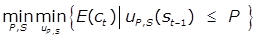The problem is resolved by calculating the P and S values that minimize the expected cost E(ct) when the optimal policy uP,S is used. To calculate the optimal manufacturing policy for fixed P and S values, the following problem must be resolved: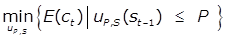Calculating the expected cost will be more or less complicated depending on the behavior of returns. If returns form a succession of independent random variables that are also independent of demand, the problem becomes considerably simpler. As we have mentioned in the introduction, it is not surprising thus that the assumption that returns are independent of demand is common in literature.

In order to make the problem computationally tractable, we proceed as follows:

·        We calculate the optimal capacities and the optimal cost assuming that: a) returns form a succession of independent random variables with a known probability distribution, and b) the probability distribution of returns is calculated according to the useful life of the products. A system that satisfies these assumptions is an approximation of the system described in section 2. In section 3 we describe how the optimal capacities are calculated.

·        We calculate the expected cost of the system described below using the capacities and the manufacturing policy obtained in the first step. The expected cost is calculated by simulation.

·        We compare the costs obtained in the steps 1 and 2. With this comparison we assess the influence of the succession independence assumption (rt) on the result.

# 3      Calculating optimal manufacturing and storage capacities

Let us assume that returns (rt) form a sequence of integer-valued random variables independent and identically distributed with probability distribution qr = p(rt = r), r = 0,…,(T2 T1 + 1)·D. By fixing P and S, we can see that the problem of calculating the optimal policy is a Markov decision problem with an infinite horizon and average reward criterion, and an optimization criterion consisting of minimizing the expected remuneration value.

The state in period t is determined by st-1, the state space is {0,1,…,S}, the actions in each period are defined by the manufacturing quantity ut, the set of actions is {0,1,…,min(P,S)}, and the remuneration is related to the cost incurred in a given period, and is equal to – (ct - Cp(P) - Cs(S)). The negative sign converts the cost function into a remuneration function; we subtract capacity costs from the cost per period to obtain a simpler expression of the remuneration function.

To define the Markov decision problem, we need to determine pij(u), the probability of transition between states i and j when decision u is taken. In other words pij(u) = p(st = j | st-1 = i, ut = u) with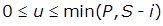. This is done in the next subsection.

## 3.1     The transition probability

In the section 2, we saw that the variable state st was dependent on st-1 and the random variables dt and rt. This dependence can be expressed as:

st = max(0, st-1 + ut - dt) + min(S – max(0, st-1 + ut - dt), rt)

Therefore, the probability of transition between states is expressed by:Where the domains Wi+u,j contain the values (d,r) such that starting from state i and taking decision u, we progress to state j. In other words, if we make k = i+u, we define the domains as follows: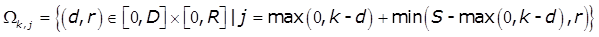For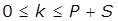and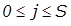. To calculate the domains Wi+u,j , we distinguish between 3 cases:

Case 1: j < S and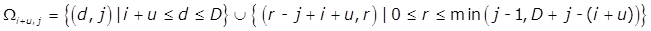Case 2: j < S andCase 3: j = S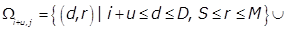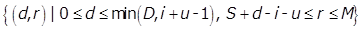Where M = (T2-T1+1)·D. Hence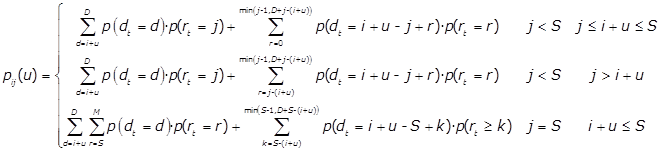Note that pij(u) is equal to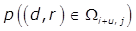, the probability that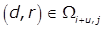.

## 3.2     State transition cost

State transition costs will be the expected value of the costs of each of the possible paths towards the transitions. Given manufacturing capacities P and storage capacities S, we want to calculate cij(u): the expected cost of the transition from state i to j when decision u is taken, i.e. cij(u) = E(c | i,j,u) where c = ct - Cp(P) - Cs(S).

Defining

c(i,j,u,d,r) = cp·u + cr·min(S – max(0, i + u - d), r) + e·max(0, r - vt,) + h·j + cec·max(0, d - i - u) + f·max(0, min(1, u))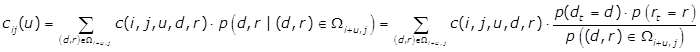Hence,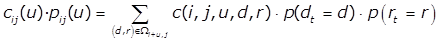Let us distinguish between 3 cases:

Case 1a: j < S and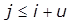: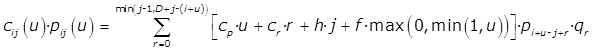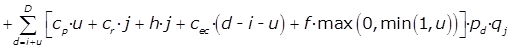Case 1b: j < S and j > i + u: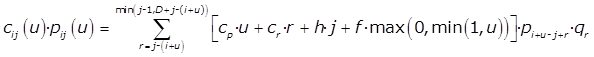Case 2: j = S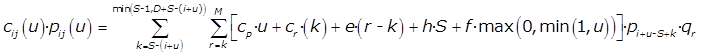+## 3.3     Calculating the optimal manufacturing policy

For each manufacturing capacity P and storage capacity S, the optimal policy is calculated by resolving the following linear program (Puterman, 1994, p. 391 ss.):

[MIN]s.t.: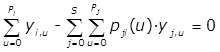i = 0,…,S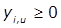i = 0,…,S, u=0,…,Pi

where Pi = min(P,S-i), yi,u are the variables, and ci(u) is: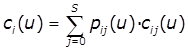If y*i,u is a basic optimal solution for the previous linear program, the optimal policy in state i will be to produce u if y*i,u > 0 and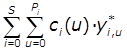is the expected cost of applying the above optimal policy. Therefore, the expected cost incurred in a period when the optimal policy is applied is

CO(P,S) = Cp(P) + Cs(S) +## 3.4     Calculating optimal capacities

In the previous section, we described how to calculate the optimal policy and obtain the expected cost when this policy is applied with fixed manufacturing and storage capacities P and S. We also defined the function CO(P,S) which at each (P,S) point takes the expected cost value on applying the optimal policy when manufacturing capacity is P and storage capacity is S. The optimal capacities in this case will be those that minimize the function CO(P,S).

Given that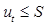and that Cp(P) is an increasing function, the optimal value is achieved for a value of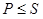. Let S* be the optimal storage capacity, then S* is bounded. Indeed, we know from (1), that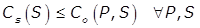In particular, for optimal manufacturing and storage capacities (P* and S*, respectively),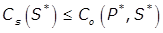We calculate CO(P0,S0) for some (P0,S0) and calculate SMAX such that Cs(SMAX) = CO(P0,S0). SMAX exists as Cs(S) will reach the value of CO(P0,S0). If not, Cs(S) would be a bounded function. This gives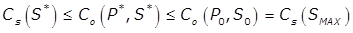And therefore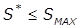as Cs(S) is a non-decreasing function.

# 4      Numerical example

In the following example, we study the optimal storage and manufacturing capacities and the optimal cost when there are variations in cp (cost per unit produced) and h (holding cost). The following parameters are used:

D = 8; p = (0, 0, 0.05, 0.1, 0.2, 0.3, 0.35)

T1 = 1, T2 = 3;  p  = (0.15, 0.25, 0.60), r = 0.7

cr = 5, e = 10, f = 25 , cr = 5, cec = 30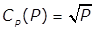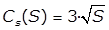We took 10 values for cp (5, 6, …, 15) and 10 values for h (0, 1, …, 10). For each pair (cp,h), we follow the procedure described in section 2.1: we calculate the optimal cost and the optimal capacities in the approximated system, we calculate the expected cost of the system described in section 2, and we compare the costs obtained in the previous steps.

## 4.1     Calculating the optimal cost in the approximated system

Table 1 shows the optimal capacities and the optimal cost calculated for each pair (cp,h) in the approximated system.

In the system described in section 2, the random variables rt (t = 1,2,…) form a succession of random variables that are dependent on demand and on each other.

We obtain the approximated model by a) relaxing this fact and assuming that returns form a succession of independent random variables, and b) calculating the probability distribution of rt following the appendix. Figure 1 shows the probability distribution of returns, qr, for the example.

 h cp = 5 cp = 6 cp = 7 cp = 8 cp = 9 S* P* Cost S* P* Cost S* P* Cost S* P* Cost S* P* Cost 0 16 10 49,1 16 10 50,9 16 10 52,7 16 10 54,5 16 10 56,3 1 12 7 57,6 12 7 59,4 12 7 61,1 12 7 62,9 12 7 64,6 2 11 6 64,7 11 6 66,5 11 6 68,2 11 6 70,0 11 6 71,7 3 10 6 71,3 10 6 73,1 10 5 74,8 10 5 76,6 10 6 78,2 4 8 6 77,3 8 6 78,9 8 6 80,5 8 6 82,1 9 6 83,7 5 7 6 82,2 7 6 83,9 8 6 85,5 8 6 87,1 8 6 88,7 6 7 6 87,0 7 6 88,7 7 6 90,4 7 6 92,0 8 5 93,7 7 7 6 91,7 7 6 93,4 7 6 95,1 7 5 96,8 7 5 98,5 8 7 6 96,5 7 6 98,2 7 6 99,9 7 5 101,6 7 5 103,3 9 7 6 101,3 7 6 103,0 7 5 104,6 7 5 106,3 7 5 108,0 10 7 6 106,0 7 5 107,7 7 5 109,4 7 5 111,1 7 5 112,8 h cp = 10 cp = 11 cp = 12 cp = 13 cp = 14 cp = 15 S* P* Cost S* P* Cost S* P* Cost S* P* Cost S* P* Cost S* P* Cost 0 16 10 58,0 17 10 59,8 17 10 61,6 17 10 63,3 17 10 65,1 16 10 66,9 1 13 7 66,4 13 7 68,1 13 7 69,8 13 7 71,5 13 7 73,3 13 7 75,0 2 11 6 73,5 11 6 75,2 11 6 76,9 11 6 78,5 11 6 80,1 11 6 81,7 3 10 6 79,8 10 6 81,4 10 6 83,0 10 6 84,5 10 5 86,1 10 5 87,6 4 9 6 85,3 9 6 86,8 9 5 88,4 9 5 89,9 9 5 91,4 9 5 93,0 5 8 5 90,3 8 5 91,9 9 5 93,5 9 5 95,0 9 5 96,6 9 5 98,1 6 8 5 95,3 8 5 96,9 8 5 98,5 8 5 100,1 8 5 101,7 8 5 103,2 7 7 5 100,2 7 5 101,9 8 5 103,5 8 5 105,1 8 5 106,6 8 5 107,9 8 7 5 104,9 7 5 106,6 7 5 108,3 7 5 110,0 7 5 111,4 7 5 112,7 9 7 5 109,7 7 5 111,4 7 5 113,1 7 5 114,7 7 5 116,0 7 5 117,3 10 7 5 114,4 7 5 116,1 7 5 117,8 7 5 119,3 7 5 120,6 7 5 121,8

Table 1. Optimal storage and manufacturing capacities, and optimal cost for each pair (cp, h) calculated following section 3, using the parameters of the example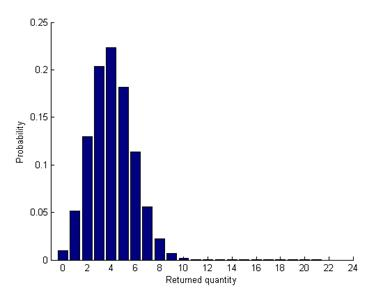Figure 1. Probability distribution for returned quantity in the example

## 4.2     Calculating the expected cost by simulation

For each pair (cp, h), we have simulated 100 times the expected cost of the system described in section 2. We have used the parameters of the example and the optimal capacities and the optimal policy calculated in section 4.1. Each expected cost were obtained by simulating the functioning of the system for 30 periods and calculating the average cost for the following 3,000 periods. In all of the cases, T1 = 1. We have performed a Lilliefors test with level of significance of 5% to test the null hypothesis that the samples comes from a distribution in the normal family’. We concluded that the hypothesis of normality is rejected in the following cases (cp, h) = {(8,0), (8,3), (10,0), (10,3), (11,3), (11,4), (13,0), (13,9), (14,8)}.

## 4.3     Comparison between dependent and independent returns

For each (cp, h) with normally distributed samples, we have performed a hypothesis test where the null hypothesis is “optimal cost calculated in section 4.1 is equal to mean value of the samples obtained in section 4.2” and the level of significance is 5%.Figure 2. Calculated cost showed in Table 1. The dots correspond to (cp, h) pairs where the difference between calculated and expected costs is not statistically significant

Figure 2 shows the optimal cost in the approximated system and the (cp, h) pairs where the difference between calculated and expected costs (obtained by simulation) is not statistically significant. Figure 2 also shows the values (cp, h) where the difference between both costs is statistically significant.

We can view in Figure 3 the differences between calculated cost (based on the return independence assumption and showed in Table 1) and estimated cost by simulation (mean value of the 100 samples).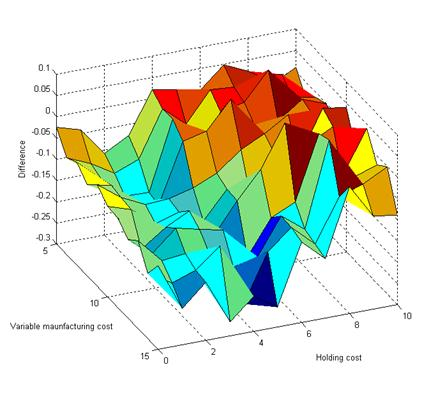Figure 3. Differences between costs, calculated as explained in section 3 for the approximated system and the simulated cost calculated in the system described in section 2

# 5      Conclusions

In this paper we have developed a model of a system with reverse logistics, stochastic demand and returns, and limited manufacturing and storage capacities. Using a linear program we have calculated the optimal manufacturing policy when capacities are fixed and we have described the way to obtain the optimal capacities.

We have described the following procedure to assess the influence of the hypothesis that returns are stochastically independent on the optimal production capacities:

·        Calculate the probability distribution of returns using the appendix.

·        Calculate the optimal policy, the optimal capacities, and the optimal cost for an approximated model following section 3.

·        Obtain samples of the expected cost of the system by simulation.

·        Perform a hypothesis test for the claim ‘the cost obtained in step 2 is equal to the cost obtained in step 3’.

·        When the claim of step 4 is rejected, conclude that the hypothesis that returns are stochastically independent is influencing on the optimal production capacities.

We have illustrated how to use this procedure with a numerical example. We concluded that the influence of the assumption that returns are stochastically independent is not significant in some cases. Indeed, the calculations are simplified considerably.

We have studied when the independence assumption is affecting the optimal capacities depending on the manufacturing and holding costs.

The findings of this study could be used as a starting point for future works. We can use the procedure described above for studying production systems, such as:

·        Study in detail the influence of the independence assumption depending on the value of remanufacturing cost and the return rate r.

·        Analyze the influence of the hypothesis that the demand and the returns are stochastically independent in models with bounded remanufacturing capacity, backorders and manufacturing and remanufacturing lead times.

## Appendix: Probability distribution of returns

We compute the probability distribution of returns in the period t, based on the probability distribution of the useful life of the product and the return rate r. To do this, we define the random variables Zt,t : units returned in period t sold in period t-t (t = T1,...T2) and define the following probability distributions related to these random variables:

Distribution of probability of Zt,t: htk = p(Zt,t = k) k = 0,…,D.

Distributions of probability of Zt,t  conditioned by dt = i (i = 0,...,D): given i we define utik = p(Zt,t = k | dt = i) (t = T1,...T2, k = 0,…,D).

We first calculated utik, the conditioned probability distributions. We know that a product’s useful life has a random duration of between T1 and T2 and once this has come to an end, the product has a probability r of being returned. Therefore, if the sales in a period are i, the probability distribution of returns they generate is: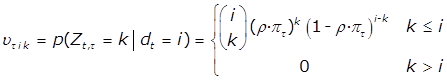For i = 0,...,D and t = T1,...T2. We have the values: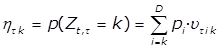for k = 0,…,D y t = T1,...T2.

We are now able to calculate the probability distribution of returns as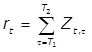, where the distribution is obtained from the probabilities of Zt,t, as. Hence,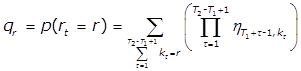for r = 0,…,(T2-T1+1)·D

This expression can be calculated through the convolution of the Zt probability distributions, with the following recurrence relationship: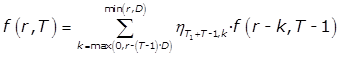for T >1 and r = 0,…,T·D:

This allows us to calculate qr = f(r, T2-T1+1) from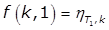k = 0,…,D.

#### References

Atasu, A., Guide, V.D.R., & Van Wassenhove, L.N. (2008). Product reuse economics in closed-loop supply chain research. Production and Operations Management, 17(5), 483-496. http://dx.doi.org/10.3401/poms.1080.0051

Fleischmann, M., & Kuik, R. (2003). On optimal inventory control with independent stochastic item returns. European Journal of Operational Research, 151, 25-37. http://dx.doi.org/10.1016/S0377-2217(02)00592-1

Fleischmann, M., Kuik, R., & Dekker, R. (2002). Controlling inventories with stochastic item returns: A basic model. European Journal of Operational Research, 138, 63-75. http://dx.doi.org/10.1016/S0377-2217(01)00100-X

Georgiadis, P., Vlachos, D., & Tagaras, G. (2006). The impact of product lifecycle on capacity of closed-loop supply chains with remanufacturing. Production and Operations Management, 15(4), 514-527. http://dx.doi.org/10.1111/j.1937-5956.2006.tb00160.x

Hax, A.C., & Candea, D. (1984). Production and Inventory Management. Englewood Cliffs, New Jersey: Prentice-Hall, Inc.

Inderfurth, K. (2004). Optimal policies in hybrid manufacturing/remanufacturing systems with product substitution. International Journal of Production Economics, 90, 325-343. http://dx.doi.org/10.1016/S0925-5273(02)00470-X

Kannan, G., Noorul Haq, A., & Devika, M. (2009). Analysis of closed loop supply chain using genetic algorithm and particle swarm optimisation. International Journal of Production Research, 47, 1175-1200. http://dx.doi.org/10.1080/00207540701543585

Kiesmüller, G.P. & van der Laan, E. (2001). An inventory model with dependent product demands and returns. International Journal of Production Economics, 72, 73-87. http://dx.doi.org/10.1016/S0925-5273(00)00080-3

Luss, H. (1982). Operations research and capacity expansion problems: A survey. Operational Reearchs, 30(5), 907-947.

Puterman, M.L. (1994). Markov decision processes: Discrete stochastic dynamic programming. New York: John Wiley & Sons, cop. 1994.

Rubio, S., Chamorro, A., & Miranda, F.J. (2008). Characteristics of the research on reverse logistics (1995-2005). International Journal of Production Research, 46(4), 1099-1120. http://dx.doi.org/10.1080/00207540600943977

Serrato, M.A., Ryan, S.M., & Gaytán, J. (2007). A Markov decision model to evaluate outsourcing in reverse logistics. International Journal of Production Research, 45, 4289-4315. http://dx.doi.org/10.1080/00207540701450161

Van der Laan, E. (2003). An NPV and AC analysis of a stochastic inventory system with joint manufacturing and remanufacturing. International Journal of Production Economics, 81-82, 317-331. http://dx.doi.org/10.1016/S0925-5273(02)00297-9

Van der Laan, E., & Salomon, M. (1997). Production planning and inventory control with remanufacturing and disposal. European Journal of Operational Research, 102, 264-278. http://dx.doi.org/10.1016/S0377-2217(97)00108-2

Van Mieghem, J.A. (2003). Capacity management, investment and hedging: Review and recent developments. Manufacturing & Service Operations Management, 5(4), 269-302. http://dx.doi.org/10.1287/msom.5.4.269.24882

Wu, S.D., Erkoc, M., & Karabuk, S. (2005). Managing capacity in the high-tech industry: A review of literature. The Engineering Economist, 50, 125-158. http://dx.doi.org/10.1080/00137910590949887

Journal of Industrial Engineering and Management, 2011

Article's contents are provided on a Attribution-Non Commercial 3.0 Creative commons license. Readers are allowed to copy, distribute and communicate article's contents, provided the author's and Journal of Industrial Engineering and Management's names are included. It must not be used for commercial purposes. To see the complete license contents, please